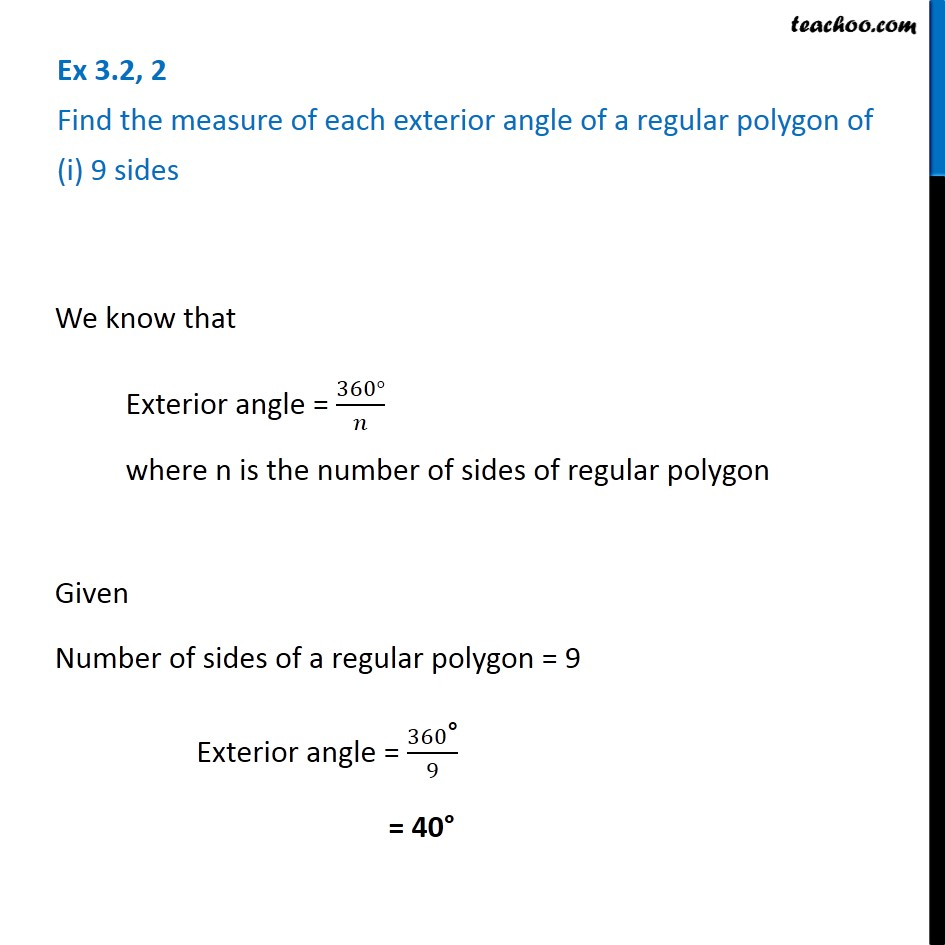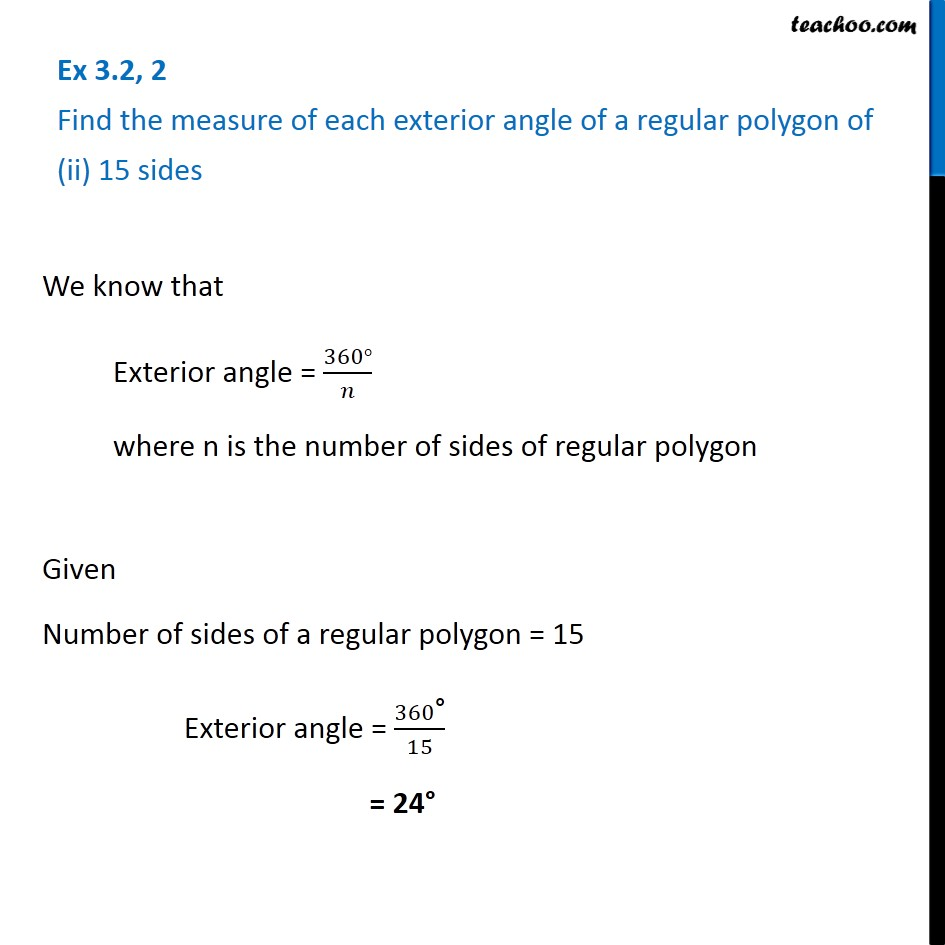Subscribe to our Youtube Channel - https://you.tube/teachoo

1. Chapter 3 Class 8 Understanding Quadrilaterals
2. Concept wise
3. Sum of Exterior Angles of Polygons

Transcript

Ex 3.2, 2 Find the measure of each exterior angle of a regular polygon of (i) 9 sidesWe know that Exterior angle = (360°)/𝑛 where n is the number of sides of regular polygon Given Number of sides of a regular polygon = 9 Exterior angle = 360"°" /9 = 40° Ex 3.2, 2 Find the measure of each exterior angle of a regular polygon of (ii) 15 sidesWe know that Exterior angle = (360°)/𝑛 where n is the number of sides of regular polygon Given Number of sides of a regular polygon = 15 Exterior angle = 360"°" /15 = 24°

Sum of Exterior Angles of Polygons